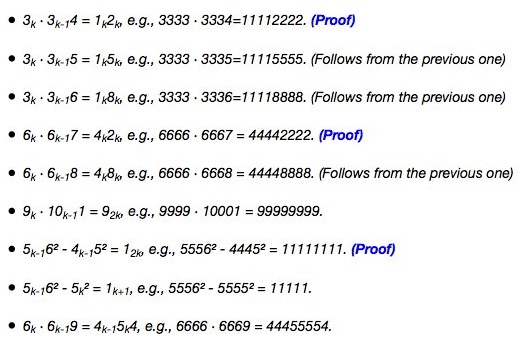# Curious Identities Involving Integer Products

This is a continuation of the page where I started to collect identities with expressions that include repeated identical digits. To make describing such numbers a manageable undertaking, I'll use subscripts to denote the number of repetitions of a digit, e.g., 1532 will denote 1111133, i.e., five 1s followed by 2 3s. In general dn means a sequence of n d's.

The identities evolved from CSIRO newsLetter, MathByEmail. An early morning exchange on twitter.com with Gary Davis and subsequent comments by James Tanton, who has observed that the repunits (the integers 111..11) with an even number of 1s are represented by the difference of squares of which one is in the form 555...56 in two ways.

• 3k · 3k-14 = 1k2k, e.g., 3333 · 3334=11112222. (Proof)

• 3k · 3k-15 = 1k5k, e.g., 3333 · 3335=11115555. (Follows from the previous one)

• 3k · 3k-16 = 1k8k, e.g., 3333 · 3336=11118888. (Follows from the previous one)

• 6k · 6k-17 = 4k2k, e.g., 6666 · 6667 = 44442222. (Proof)

• 6k · 6k-18 = 4k8k, e.g., 6666 · 6668 = 44448888. (Follows from the previous one)

• 9k · 10k-11 = 92k, e.g., 9999 · 10001 = 99999999.

• 5k-16² - 4k-15² = 12k, e.g., 5556² - 4445² = 11111111. (Proof)

• 5k-16² - 5k² = 1k+1, e.g., 5556² - 5555² = 11111.

• 6k · 6k-19 = 4k-15k4, e.g., 6666 · 6669 = 44455554.

Subscripts can be easily converted to decimal notations. Observe that

1t = 9t / 9 = (10t - 1) / 9.

So, for example,

3k5m6n7 = 7 + 10 · 6 · (10n - 1) / 9 + 10n + 1 · 5 · (10m - 1) / 9 + 10m + n + 1 · 3 · (10k - 1) / 9.### Number Curiosities3k · 3k-14 = 1k2k.

 3k · 3k-14 = 3 · (10k - 1)/9 × [3 · (10k - 1)/9 + 1] = (10k - 1)/3 × [(10k - 1)/3 + 1] = (102k - 2 · 10k + 1)/9 + (10k - 1)/3 = (102k + 10k - 2)/9 = (102k - 1)/9 + (10k - 1)/9 = 12k + 1k = 1k2k.6k · 6k-17 = 4k2k.

 6k · 6k-17 = 6(10k - 1)/9 × (6(10k - 1)/9 + 1) = 2(10k - 1)/3 × (2(10k - 1)/3 + 1) = 4(102k - 2 · 10k + 1)/9 + 2(10k - 1)/3 = 4(102k - 2 · 10k + 1)/9 + 6(10k - 1)/9 = 4 · 102k - 2 · 10k - 2)/9 = 4 · (102k - 1)/9 - 2 · (10k - 1)/9 = 42k - 2k = 4k2k

Of course it is alos possible to derive this identity from 3k · 3k-14 = 1k2k. Indeed, let's multiply the latter by 4, splitting 4 into two factors of 2 on the left:

6k · 6k-18 = 4k8k,

which is the next identity. Subtracting 6k from both sides gives

6k · 6k-17 = 4k2k,

To remind: